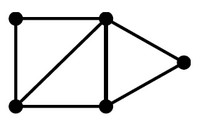# Graph - Direction

A direction is a property of an edge.

An edge is directed if it has a defined start (its source) and end (its target, also called its destination).

Recommended Pages(Network|Graph) - Directed Graph (or digraph)

A directed graph (or digraph) is a graph, where the edges have a direction associated with them. Directed edges are suitable for modeling asymmetric relations undirected Data flow modeling ...Graph - (Edge | Links | Arcs | Lines | Arrows) - Association

An edge model a relationship betweentwo node in a graph. Every edge model therefore a binary relationship (relationship between two elements) . An Edge is also known as: Links Arcs Lines Arrows...Graph - Undirected graph

A undirected graph is a graph without direction information Undirected edges are suitable for modeling symmetric relations directed graph